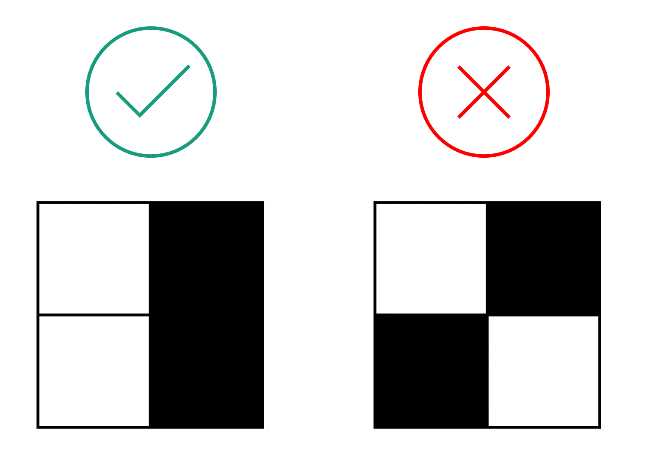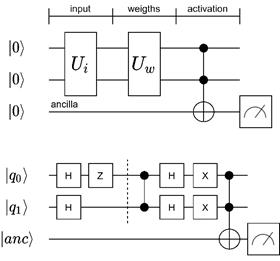# Pattern Recognition Problem

Quantum Neural Networks can be implemented in different ways and the most modern forms that fit best to our current quantum devices implement feed forward networks with back propagation as hybrid quantum classical algorithms. The case study here starts with a more simple example of a neural network: A single neuron in a perceptron-like setup is coded by quantum circuits. A modern hybrid algorithm is then introduced with QAOA in the next study.

### Introduction

Pattern recognition as one of the most common applications of machine learning is about the automatic discovery of regularities in data by a machine that uses computer algorithms for classification based on statistical information, historical data and memory. Commonly, machine learning methods for classification rely on neural networks, which contain a set of neurons that are ordered in several succeeding layers. Each of these neurons receives the results from the preceding layer as input arguments and provides an output defined by a certain activation function for the following layer. The inputs of a neuron are equipped with weights whose values are adapted by an optimization scheme during the training process, which consists in traversing training data with pre-labelled outcomes.Figure 1: A neuron taking m input arguments and delivering output based on a threshold activation

### A simple recognition problem

For a realisation of an artificial feed forward neural network on a quantum computer, we consider a simple recognition problem based on small “binary pictures”. The goal is to identify each picture with a pattern of two black pixels arranged one upon the other or side by side as illustrated by Figure 1. The considerations are based on the studies [1,2].Figure 2: Visualisation of two binary images, whereof the left one represents the searched pattern

For this task we use a network, which consists of quantum artificial neurons – implementable on the IBM superconducting quantum processors. Each of the quantum neurons provide a potential advantage in storage capacity and is able to proceed classification tasks that are impossible to do via single classical nodes only. For classification problems, quantum computing provides a potential exponential speed-up.

### Quantum neural network setup

The quantum neural network is embedded into a hybrid approach using quantum nodes, which take classical information as input and deliver a classical output. The quantum circuit for a single neuron is composed out of two encoding steps and the activation function as depicted by Figure 3.Figure 3: The circuit for a single quantum neuron with an exemplary realisation for a combination of image and weights

Since we focus on input images with four pixels, we need two qubits for the processing. An additional ancilla qubit receives and delivers the neuron’s output. Two unitary operations serve to encode the input arguments and the weights. The activation is proceeded via a multi-CNOT gate. The result can either be measured (as done in Figure 3) or passed through to further quantum neurons.

Finally, the target patterns distinguished from the other ones by delivering a probability amplitude of the stategreater that, which serves as the recognition threshold.

### Literature

 Tacchino, F., Macchiavello, C., Gerace, D., and Bajoni, D., 2019. An artificial neuron implemented on an actual quantum processor, npj Quantum Information 5, 26.

 Tacchino, F., Barkoutsos, P., Macchiavello C., Tavernelli, I., Gerace D., and Bajoni D., 2020. Quantum implementation of an artificial feed-forward neural network, Quantum Science and Technology 5, 044010.